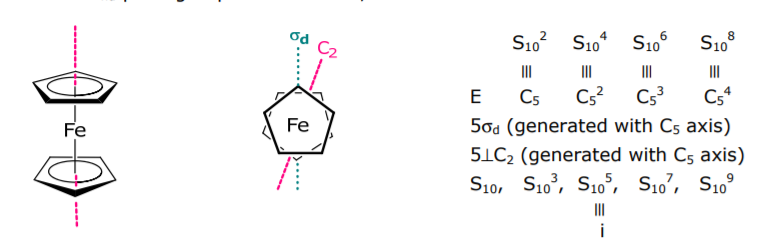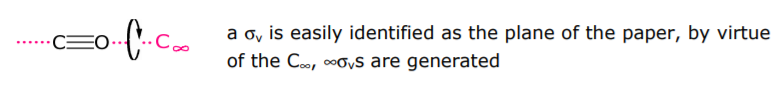# 1.5: Molecular Point Groups 2

The D point groups are distiguished from C point groups by the presence of rotation axes that are perpindicular to the principal axis of rotation.

Dn : Cn and n⊥C2 (h = 2n)

Example: Co(en)3 3+ is in the D3 point group,In identifying molecules belonging to this point group, if a Cn is present and one ⊥C2 axis is identified, then there must necessarily be (n–1)⊥C2s generated by rotation about Cn.

Dnd : Cn, n⊥C2, nσd (dihedral mirror planes bisect the ⊥C2s)

Example: allene is in the D2d point group,Two C2s bisect σds. The example on the bottom on pg 3 of the Lecture 4 notes was a harbinger of this point group. As indicated there, it is often easier to see these perpendicular C2s by reorienting the molecule along the principal axis of rotation.

Note: Dnd point groups will contain i, when n is oddDnh : Cn, n⊥C2, nσv, σh (h = 4n)C∞v : C and ∞σv (h = ∞)

linear molecules without an inversion centerD∞h : C, ∞⊥C2, ∞σv, σh, i (h = ∞)

linear molecules with an inversion centerwhen working with this point group, it is often convenient to drop to D2h and then correlate up to D∞h

Td : E, 8C3, 3C2, 6S4, 6σd (h = 24)Oh : E, 8C3, 6C2, 6C4, 3C2 (=C4 2 ), i, 6S4, 8S6, 3σh, 6σd (h = 48)O : E, 8C3, 6C2, 6C4, 3C2 (=C4 2 ) A pure rotational subgroup of Oh, contains only the Cn’s of Oh point group T : E, 8C3, 3C2 A pure rotational subgroup of Td, contains only the Cn’s of Td point groupIh : generators are C3, C5, i (h = 120) $$\Longrightarrow$$ the icosahedral point group

Kh : generators are Cφ, Cφ’, i (h = ∞) $$\Longrightarrow$$ the spherical point group

Flow chart for assigning molecular point groups: